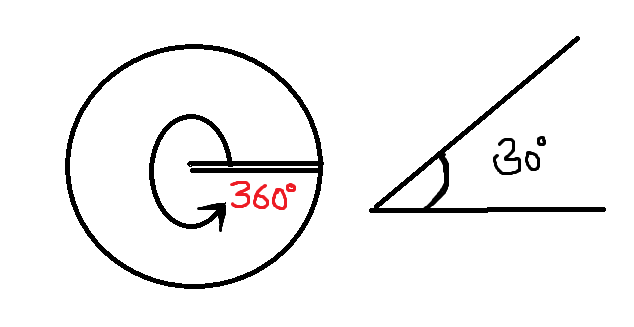﻿ Angle Converter - FREE Unit Converter

### To :

Decimals
ANGLE CONVERTER

What is Angle?

In geometry the turn measured between two straight lines sharing a common vertex is defined angle. Angle is measured in Degrees. ( ° )

We use a little circle ° following the number to mean degrees.

In case of very accurate measurement we just add decimal places to the degrees, For example 36.72°The small circle after the number means "degrees". So the above would be pronounced "thirty six point seven two degrees"

There are 360 degrees in one Full Rotation (one complete circle around).

Full circle: 360 degrees

Half Circle : 180 degrees (STRAIGHT ANGLE)

Quarter of a circle :90° (RIGHT ANGLE)Common units to measure Angle:

• Degree